# Dividing decimals multiplying decimals

## Multiplying decimals

To multiply two decimals, gotta:
1. perform the multiplication, ignoring the commas;
2. separate with a comma as many figures on the right, how many of them after the decimal point in both factors together.

Multiply fractions of 13.2% and 0.2. After completing the multiplication, ignoring the commas, get: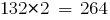. Separate decimal point right as many digits as is the decimal point in both factors together, that is two digits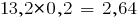.

Consider other examples of multiplication of decimal numbers: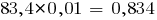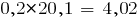### Multiplying decimals and integers

The product of decimals and natural numbers is called a sum of terms, each of which is equal to the given decimal, and the number of summands is equal to this positive integer.

To multiply a decimal fraction by a natural number, necessary:
1. multiply it by that number, ignoring the comma;
2. in the resulting product to separate the decimal point as many digits to the right, how many of them separated by a comma in decimal fractions.

### Multiplying decimals by 10, 100, 1000, etc.

To multiply a decimal fraction by 10,100,1000, etc. need this fraction to move the decimal point that many digits to the right, how many zeros is in the multiplier after one.

Example: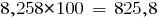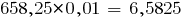If the result is fewer digits than necessary to separate with a comma, ahead write zero or several zeros.## Dividing decimals

To divide a decimal fraction by a natural number, gotta:
1. to divide a fraction by that number, ignoring the comma;
2. to deliver to private a comma, when the division of the integer part.

### Division by a decimal

Division by decimals is replaced with division by a natural number.

To divide a number by a decimal, gotta:
1) and divided by the divider to move the decimal point right as many digits,
how many of them after the decimal point in the divisor;
2) then perform a division by a natural number;
3) if RMS do not have enough characters, right credited with zeros.

The rule is a consequence basic properties of fractions (hell model fraction division): the numerator and denominator of a fraction can be multiplied by a nonzero number (to expand a fraction).

In this case, multiply by 10,100,1000, etc.

For example,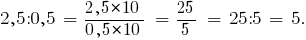In short can be written as: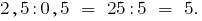Moved someone in divided by 2.5 and the divider 0.5 to so many characters, how many of them after the decimal point in the divisor is 0.5, that is, one character.

### Dividing decimals by 0,1; 0,01; 0,001, etc.

To divide decimals by 0,1; 0,01; 0,001 etc., should be moved to this point as many figures to the right, how many zeros comes before one in the divisor (or multiply the dividend and the divisor by 10, 100, 1000і etc.). If digits is not enough, first you have to ascribe at the end of a decimal, the zeros (as needed).

Consider the examples of dividing by 0,1; 0,01; 0,001, by applying the rule of division by a decimal:

• in divided by the divisor and move the decimal to the right by as many digits,
how many of them after the decimal point in the divisor;
• then perform the division by a natural number.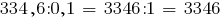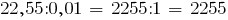Tags:
Chapter:
Versions in other languages:
Share with friends: